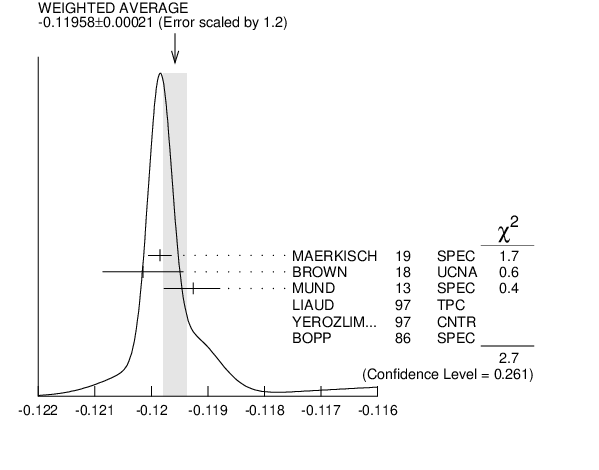# ${{\boldsymbol n}}$ $\rightarrow$ ${{\boldsymbol p}}{{\boldsymbol e}^{-}}{{\overline{\boldsymbol \nu}}_{{e}}}$ DECAY PARAMETERS

See the above Note on Baryon Decay Parameters.'' For discussions of recent results, see the references cited at the beginning of the section on the neutron mean life. For discussions of the values of the weak coupling constants ${\mathit g}_{{{\mathit A}}}$ and ${\mathit g}_{{{\mathit V}}}$ obtained using the neutron lifetime and asymmetry parameter$~\mathit A$, comparisons with other methods of obtaining these constants, and implications for particle physics and for astrophysics, see DUBBERS 1991 and WOOLCOCK 1991 . For tests of the $\mathit V−\mathit A$ theory of neutron decay, see EROZOLIMSKII 1991B, MOSTOVOI 1996 , NICO 2005 , SEVERIJNS 2006 , and ABELE 2008 .

# ${{\boldsymbol e}^{-}}$ ASYMMETRY PARAMETER $\boldsymbol A$ INSPIRE search

This is the neutron-spin electron-momentum correlation coefficient. Unless otherwise noted, the values are corrected for radiative effects and weak magnetism. In the Standard Model, $\mathit A$ is related to $\lambda {}\equiv\mathit g_{A}/\mathit g_{V}$ by $\mathit A$ = $-2$ $\lambda$ ($\lambda$ + 1) $/$ (1 + 3$\lambda {}^{2}$); this assumes that $\mathit g_{A}$ and $\mathit g_{V}$ are real.
VALUE DOCUMENT ID TECN  COMMENT
$\bf{ -0.1187 \pm0.0010}$ OUR AVERAGE  Error includes scale factor of 2.6.
$-0.12015$ $\pm0.00034$ $\pm0.00063$ 1
 2018
UCNA Ultracold ${{\mathit n}}$, polarized
$-0.11926$ $\pm0.00031$ ${}^{+0.00036}_{-0.00042}$ 2
 2013
SPEC Cold ${{\mathit n}}$, polarized
$-0.1160$ $\pm0.0009$ $\pm0.0012$
 1997
TPC Cold ${{\mathit n}}$, polarized
$-0.1135$ $\pm0.0014$ 3
 1997
CNTR Cold ${{\mathit n}}$, polarized
$-0.1146$ $\pm0.0019$
 1986
SPEC Cold ${{\mathit n}}$, polarized
• • • We do not use the following data for averages, fits, limits, etc. • • •
$-0.11952$ $\pm0.00110$ 4
 2013
UCNA See BROWN 2018
$-0.11966$ $\pm0.00089$ ${}^{+0.00123}_{-0.00140}$ 5
 2012
UCNA
$-0.11966$ $\pm0.00089$ ${}^{+0.00123}_{-0.00140}$
 2010
UCNA See PLASTER 2012
$-0.1138$ $\pm0.0046$ $\pm0.0021$
 2009
SPEC Ultracold ${{\mathit n}}$, polarized
$-0.1189$ $\pm0.0007$ 6
 2002
SPEC See MUND 2013
$-0.1168$ $\pm0.0017$ 7
 2001
CNTR Inferred
$-0.1189$ $\pm0.0012$
 1997 D
SPEC Cold ${{\mathit n}}$, polarized
$-0.1160$ $\pm0.0009$ $\pm0.0011$
 1995
TPC See LIAUD 1997
$-0.1116$ $\pm0.0014$
 1991
CNTR
$-0.114$ $\pm0.005$ 8
 1979
CNTR Cold ${{\mathit n}}$, polarized
$-0.113$ $\pm0.006$ 8
 1975
CNTR Cold ${{\mathit n}}$, polarized
1  BROWN 2018 gets $\mathit A$ = $-0.12054$ $\pm0.00044$ $\pm0.00068$ and $\lambda$ = $-1.2783$ $\pm0.0022$. We quote the combined values that include the earlier UCNA measurements (MENDENHALL 2013 ).
2  This MUND 2013 value includes earlier PERKEO II measurements (ABELE 2002 and ABELE 1997D), with a correction to those results.
3  YEROZOLIMSKY 1997 makes a correction to the EROZOLIMSKII 1991 value.
4  MENDENHALL 2013 gets $\mathit A$ = $-0.11954$ $\pm0.00055$ $\pm0.00098$ and $\lambda$ = $-1.2756$ $\pm0.0030$. We quote the nearly identical values that include the earlier UCNA measurement (PLASTER 2012 ), with a correction to that result.
5  This PLASTER 2012 value is identical with that given in LIU 2010 , but the experiment is now described in detail.
6  This is the combined result of ABELE 2002 and ABELE 1997D.
7  MOSTOVOI 2001 calculates this from its measurement of $\lambda =\mathit g_{\mathit A}/\mathit g_{\mathit V}$ above.
8  These results are not corrected for radiative effects and weak magnetism, but the corrections are small compared to the errors.${{\mathit e}^{-}}$ asymmetry parameter $\mathit A$
References:
 BROWN 2018
PR C97 035505 New result for the neutron $\beta$-asymmetry parameter $A_0$ from UCNA
 MENDENHALL 2013
PR C87 032501 Precision Measurement of the Neutron Beta-Decay Asymmetry
 MUND 2013
PRL 110 172502 Determination of the Weak Axial Vector Coupling $\mathit \lambda$ = $\mathit g_{A}/\mathit g_{V}$ from a Measurement of the ${{\mathit \beta}}$-Asymmetry Parameter $\mathit A$ in Neutron Beta Decay
 PLASTER 2012
PR C86 055501 Measurement of the Neutron ${{\mathit \beta}}$-Asymmetry Parameter ${{\mathit A}^{0}}$ with Ultracold Neutrons
 LIU 2010
PRL 105 181803 Determination of the Axial-Vector Weak Coupling Constant with Ultracold Neutrons
 PATTIE 2009
PRL 102 012301 First Measurement of the Neutron $\beta$ Asymmetry with Ultracold Neutrons
 ABELE 2002
PRL 88 211801 Is the Unitarity of the Quark Mixing CKM Matrix Violated in Neutron $\beta$ Decay?
 MOSTOVOI 2001
PAN 64 1955 Experimental Value of $\mathit G_{a}/G_{v}$ from a Measurement of Both $\mathit P$-odd Correlations in Free-neutron Decay
 ABELE 1997D
PL B407 212 A Measurement of the $\beta$ Asymmetry $\mathit A$ in the Decay of Free Neutrons
 LIAUD 1997
NP A612 53 The Measurement of the $\beta$ Asymmetry in the Decay of Polarized Neutrons
 YEROZOLIMSKY 1997
PL B412 240 Corrigendum: Corrected Value of the $\beta$ Emission Asymmetry in the Decay of Polarized Neutrons Measured in 1990
 SCHRECKENBACH 1995
PL B349 427 A New Measurements of the $\beta$ Emission Asymmetry in Free Decay of Polarized Neutrons
 EROZOLIMSKII 1991
PL B263 33 New Measurement of the Electron Neutron Spin Asymmetry in Neutron $\beta$ Decay
 BOPP 1986
PRL 56 919 The $\beta$ Decay Asymmetry of the Neutron and $\mathit g_{a}$/g$_{v}$
 EROZOLIMSKII 1979
SJNP 30 356 Measurement of the Spin Electron Correlation Coefficient in the Decay of Polarized Neutrons and the Determination of the $\mathit g_{a}$/g$_{v}$ Ratio
 KROHN 1975
PL 55B 175 $\mathit G_{A}/G_{V}$ Measured in the Decay of Polarized Neutrons